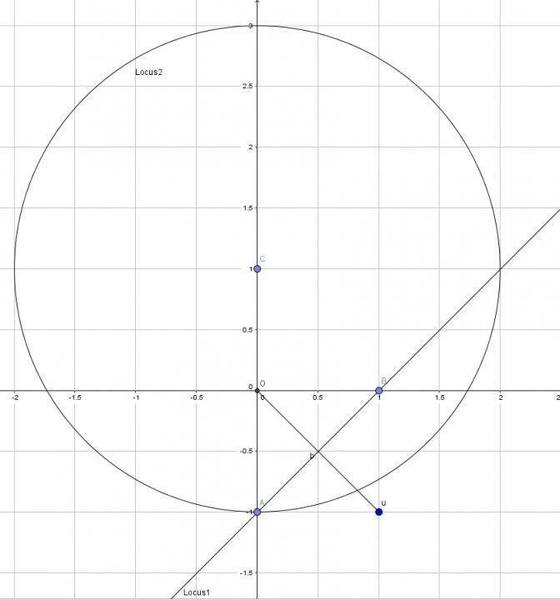# Find the argument of the complex numbers

• chwala

Gold Member

## Homework Statement

a) The complex number ## 1-i ## is denoted by ##u##. On an argand diagram, sketch the loci representing the complex numbers ## z## satisfying the equations ## |z-u|= |z| and |z-i|=2 ##
b) Find the argument of the complex numbers represented by the points of intersection of the two loci above.

## The Attempt at a Solution

a)The points on the argand diagram are ## (1,-i), (0,i)## now for ## |z-u|= |z| ##, the textbook indicates that we join point## (1,-i) ## to the origin and draw a bisector of the line segment, why? for point ## (0,i),## this point will have a radius of 2 units and the loci will be a circle, for this one i understand.
b)Now the points of intersection seem to be the point ## (2,i)## which gives an angle of ##θ=26.56^0 ## which is correct, and the other intersection point will give ## θ= 270^0 ## or ##-0.5Π##, i don't understand how the second argument of ## θ= 270^0## is found. regards

Hello Chwala,

##|z−u|=|z|##
with ##u## known is an equation for complex number ##z##. What does this give you when you work it out (write it out completetly in real part and imaginary part of ##z##) ?

 unclear and typo: I mean ##|c|^2 = c^*\,c \ ## with ##c^*## the complex conjugate of ##c##.

Last edited:
Is this correct
##(z-u)^2=z^2 ##
##(z-1+i)(z-1+i)= z^2##
##z^2-2z+2iz-2i=z^2##
##-2z+2iz-2i=0##
##z(-2+2i)=2i##
##z=(2i/-2)+(2i/2i)##
##z=-i+1##
##z=1-i##
from here the point on argand diagram is ##(1,-1)## now how do we draw the locus, why are we joining this to the origin and then we go ahead to find the bisector of this line segment from the point ##(1,-1)## to the origin.

Last edited:
Is it correct to state that ##|z-i|=2## is equal to
##(z-i)(z-i)=4##
##z^2-2iz-5=0## how do we proceed from here? ok here i know that the center is ##(0,1)## how do we get here...good evening Bvu

ok we can say##(z-i)^2=2^2##
##(z-i)=2## centre ##(0,1)## and radius of 2 units comparing with the other one i.e ##(z-1+i)=0## centre ##(1,-1)## radius 0 units? are these statements valid

Last edited:
Is it correct to state that ##|z-i|=2## is equal to
##(z-i)(z-i)=4##
No. For a complex number ##z##, ##|z|²= \bar z z## or ##|z|²= z^* z##, where ##\bar z## and ##z^*## both denote the complex conjugate of ##z##.

sam then how do we solve## |x-2|=4?##
I think the correct step would be ##(x-2)(x-2) - 16 = 0##
Or are you saying am wrong on this? alternatively ##(x-2)=4, x=6 ##
## -(x-2)=4, x=-2##
the correct values are ##x=-2 and x=6##
then
it follows that ##|z-1|=2## is the same as ##(z-i)(z-i)=4##
kindly note that i am not saying your approach is wrong, i just want to know whether my statement is correct, if its correct can i build on it to answer the question or do i have to use your approach of ##z.z*##

sam then how do we solve## |x-2|=4?##
I think the correct step would be ##(x-2)(x-2) - 16 = 0##
Or are you saying am wrong on this? alternatively ##(x-2)=4, x=6 ##
## -(x-2)=4, x=-2##
then
If ##x## is real, then yes, this is correct.
In your previous post, ##z-i## was complex. In that case, ##|z-i|^2=(\bar z+i)(z-i)##.

it follows that ##|z-1|=2## is the same as ##(z-i)(z-i)=4##
kindly note that i am not saying your approach is wrong, i just want to know whether my statement is correct, if its correct can i build on it to answer the question or do i have to use your approach of ##z.z*##
No, ##|z-i|=2## doesn't imply that ##(z-i)(z-i)=4##.
For complex numbers with a non-zero imaginary part, ##|z|² \neq z²##.

Last edited:
ok i get you now using ## |z| = zz^* ## i am getting ## z= 1-i ,## and ##zz^*=2## is this correct?

ok i get you now using ## |z| = zz^* ## i am getting ## z= 1-i ,## and ##zz^*=2## is this correct?
Yes, ##|1-i|²=2##.

(By the way, it should be ## |z|² = zz^* ##)

For the loci .. from post 1, why are we drawing a bisector of the line segment, secondly how do we arrive at ## ∅=-0.5π=270^0##

For the loci .. from post 1, why are we drawing a bisector of the line segment, secondly how do we arrive at ## ∅=-0.5π=270^0##
You are drawing a bisector because the equation ##|z-u|= |z|## represents all the complex numbers (or points in the argand diagram) that are at the same distance from the point ##(0,0)## and the point ##u=(1,-1)##.

You may want to look at the diagram for this question:Last edited:
•BvU
Thanks Samy A , i will research more in this area, particularly sketching of the locus...how did we arrive at the point ##(0,0)##?
I think that is where i am not understanding, i already know that ##u## is the point ##(1,-1)##

Thanks Samy A , i will research more in this area, particularly sketching of the locus...how did we arrive at the point ##(0,0)##?
I think that is where i am not understanding, i already know that ##u## is the point ##(1,-1)##
We get the point ##(0,0)## because ##|z|## is equal to the distance between the complex number (or point) ##z## and the origin ##(0,0)##.
Or said differently, if ##z=(x,y), |z|²=x²+y²=(x-0)²+(y-0)²##.

Thanks Samy, now clear.